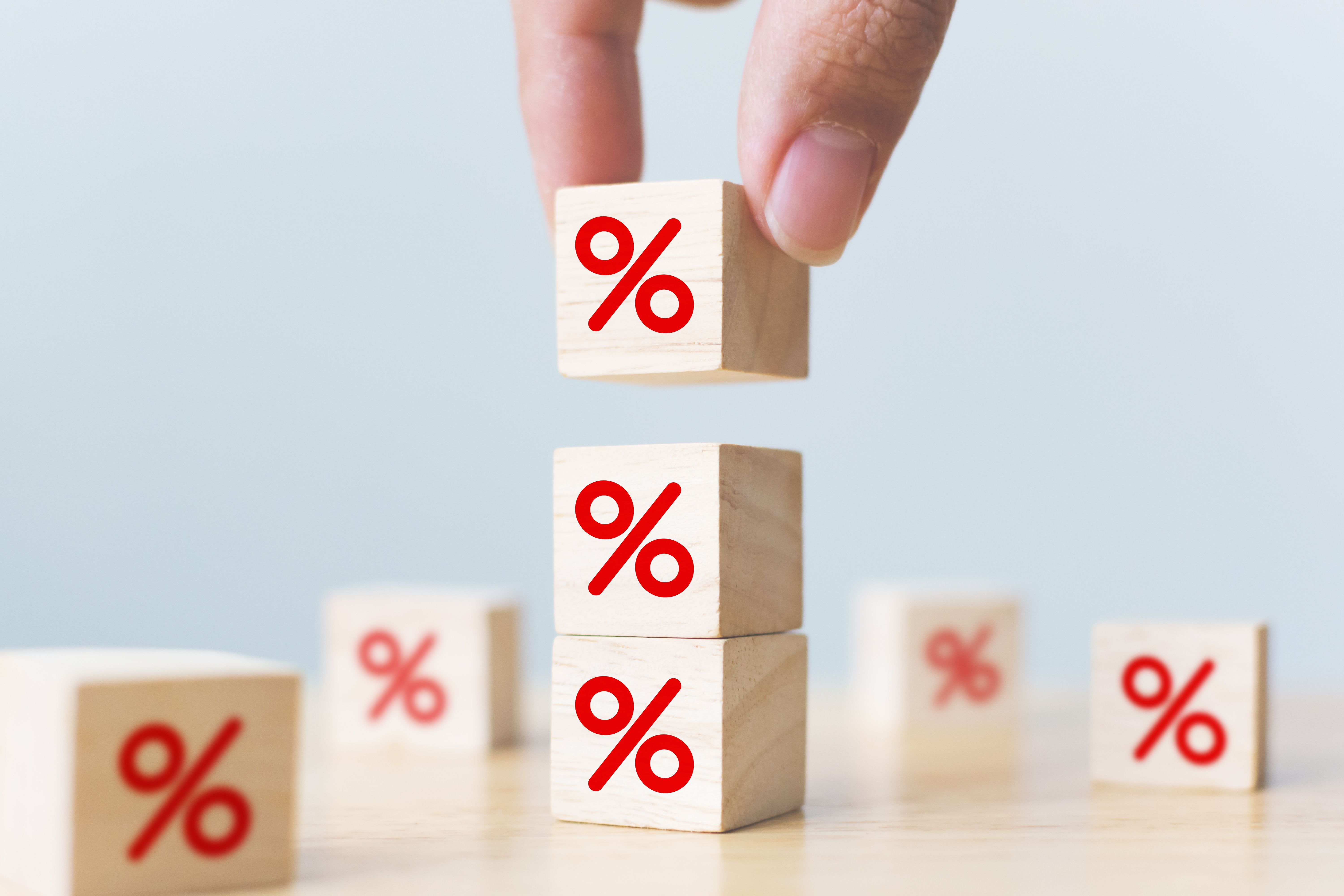# Fixed term: how much do I earn with the new rate if I deposit \$700,000?The Central Bank (BCRA) decided last month to raise the monetary policy rate (including the fixed term traditional) in 21 percentage pointsuntil the 118% (Annual Nominal Rates, TNA), which is equivalent to a 209% in effective annual terms (TEA) and an effective monthly return of 9.7%.

Regardless of the bank in which the saver makes the investment, the profit will be the same, since the rate is regulated and is the same for everyone.

## Fixed term: how much do I earn if I invest \$10,000 in 30 days?

At current interest rates, depositing \$10,000 yields a profit of almost \$970, equivalent to 9.7% of the original investment.

## Fixed term: how much do I earn if I invest \$100,000 in 30 days?

This means that if a person makes a fixed term by 30 days of \$100,000at the end of the term you will receive \$109,698.63: the \$100,000 that I had initially deposited plus a interest of \$9,698.63.

## Fixed term: how much do I earn if I invest \$500,000 in 30 days?

If \$500,000 is invested in 30 days, the profit is \$48,493.

## Fixed term: how much do I earn if I invest \$700,000 in 30 days?

With \$700,000 million invested you earn per month \$67,890which implies a good reinforcement to the pocket.

## Fixed term: how much do I earn if I invest \$1,000,000 in 30 days?

In the same sense, if you invest \$1 million in a fixed period, with the 118% ratethe 30 day profit It is \$96,986,301.

For the deposits of more than \$30 million made by companies (legal entity), the guaranteed minimum rate will be from 111%with a monthly effective interest of 9.12%.

Source: Ambito